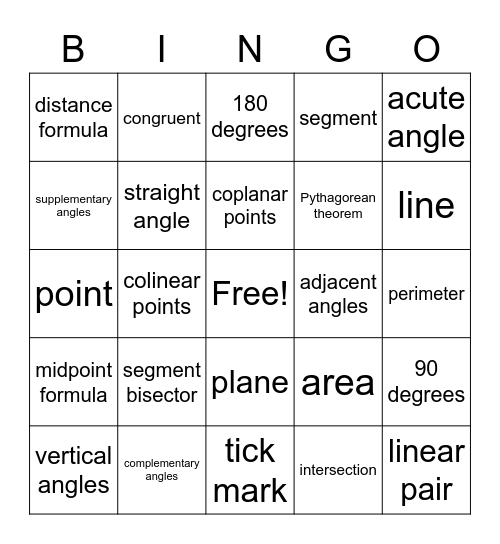# Geometry Tools BingoThis bingo card has a free space and 28 words: 90 degrees, ray, Pythagorean theorem, intersection, supplementary angles, 180 degrees, congruent, midpoint formula, adjacent angles, vertical angles, point, segment, complementary angles, tick mark, line, endpoints, segment bisector, straight angle, coplanar points, plane, distance formula, angle bisector, linear pair, colinear points, perimeter, area, acute angle and obtuse angle.

## Play Online